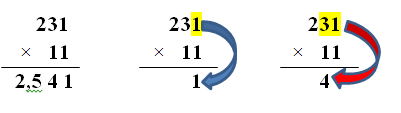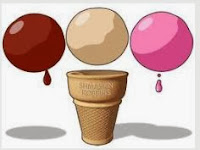### Using Bloom's Taxonomy in Geometry Class

As one of their assignments, my college students are required to create a practice test using pre-selected math vocabulary. This activity prompts them to review, look up definitions and apply the information to create ten good multiple choice questions while at the same time studying and assessing the material. Since I want the questions to be more than Level 1 (Remembering) or Level II (Understanding) of Bloom's Taxonomy, I give them the following handout to help them visualize the different levels.  My students find it to be simple, self explanatory, easy to understand and to the point.

Level I - RememberingWhat is this shape called?

Level II - Understanding

Circle the shape that is a triangle.

Level III - Applying

Enclose this circle in a square.Level IV - Analyzing

What specific shapes were used to draw the picture on your right?

Level V - Evaluating

How is the picture on your right like a real truck?  How is it  different?

Level VI - Creating

Create a new picture using five different geometric shapes. (You may use the same shape more than once, but you must use five different geometric shapes.)

As teachers, we are only limited by our imagination as to the activities we ask our students to complete to help them prepare for a test. However, we still need to teach and provide information so the students can complete these types of tasks successfully. With the aid of the above chart, my students create well written practice tests using a variety of levels of Bloom's. When the task is completed, my students have also reviewed and studied for their next math exam. I consider that as time well spent!

If you would like a copy of the above chart in a similar but more detailed format, it is available on Teachers Pay Teachers as a FREE resource.

### The Eleventh Hour? A Trick About Multiplying by 11

The mathematician magician is still here, sharing her tricks. This week it is the elevens. Before we demonstrate the trick, I have to get on my soap box for just a moment. In my humble opinion, all students should know their times tables through 12 even though the Common Core Standard for third grade says through 10 x 10. Remember, Common Core is the minimum or base line of what is to be learned. In Algebra, I insist that my students know the doubles through 25 x 25 and the square roots of those answers up to 625. It saves so much time when we are working with polynomials.

Now to our our amazing mathematical "trick". Let's look at the problem below which is 231 x 11.First we write the problem vertically. Next, we bring down the number in the ones place which in this case is a one. Now we add the digits in the ones and tens place which is 3 + 1 and get the sum of four which is brought down into the answer.Moving over to the hundreds place, we add that digit with the digit in the tens place 2 + 3 and get an answer of five which we bring down. Finally, we bring down the digit in the hundreds place which is a two. The answer to 231 x 11 is 2,541.

Now try 452 x 11 in your head. Did you get 4,972? Let's try one more. This time multiply 614 by 11. I'm waiting...... Is your answer 6,754?

Now it is time to make this process a little more difficult. What happens if we have to regroup or carry in one of these multiplication problems?We will multiply 784 by 11. Notice that we start as we did before by just bringing down the number in the ones place. Next, we add 8 + 4 and get a sum of 12. We write down the 2 but carry or regroup the one. We now add 7 + 8 which is 15 and then add in the 1 we are carrying. That makes 16. We bring down the 6 but carry the 1 over. We have a 7 in the hundreds place, but must add in the one we are carrying to get a sum of 8. Thus our answer is 8,624.

Let's see if you can do these without paper or pencil. 965 x 11 768 x 11 859 x 11 After working the problems in your head, write down your answers and check them with a calculator. Try making up some four and five digit problems because this is a non-threatening way to have your students practice their multiplication facts. Have fun!

### Anno's Mysterious Multiplying Jar - Learning About Factorials

Factorial is a word that mathematicians use to describe a special kind of numerical relationship. Factorials are very simple things. They are just products, indicated by the symbol of an exclamation mark. The factorial function (symbol: !) means to multiply a series of descending natural numbers. For instance, "five factorial" is written as "5!" (a shorthand method) and means 5 × 4 × 3 × 2 × 1 = 120. Factorials are used in determining the numbers of combinations and permutations and in finding probability.Now all of that may seem above your mathematical head, but let me introduce you to the book Anno's Mysterious Multiplying Jar by Masaichir and Mitsumasa Anno.  It is a story about one jar and what is inside it. Anno begins with the jar, which contains one island, that has two countries, each of which has three mountains. The story continues like this until 10 is reached.  The colorful pictures are arranged within borders on the page as many times as the number of objects being discussed. For instance when four walled kingdoms are introduced, four kingdoms are on the page.

The explanation of 10! in the back of the book is also very helpful. Even if children do not understand the concept being taught, they will certainly appreciate the detailed colored drawings and imaginative story! The book is best for kids who have been introduced to at least basic multiplication facts, but younger kids will enjoy counting and looking at the pictures even if the rest of it is over their heads; so, this book helps with multiplying skills as well as the mathematical concept of factorials.

You might give the students a worksheet to keep track of how many islands, rooms, etc. there are. The final question is how many jars are there. Hopefully there will some students who catch on to the factorial concept, find the pattern and discover the answer!

Here is an example of how you might use factorials in solving a word problem.  How many different arrangements can be made with the letters from the word MOVE?  Because there are four different letters and four different spaces, this is how you would solve the problem.

____   ____   ____   ____
Four Possible Spaces

All four letters could be placed in the first space. Once the first space is filled, only three letters remain to fit in the second space. Once the second space is filled with a letter, two letters remain to write in the third space. Finally, only one letter is left to take the fourth and final space. Hence, the answer is a factorial (4!) = 4 × 3 × 2 × 1 = 24 arrangements.

Try some problems in your classroom. Start with an imaginary character, Cal Q. Late, who is working at an Ice Cream Store called Flavors. A hungry customer orders a triple scoop ice cream cone with Berry, Vanilla, and Bubble Gum ice cream. How many different ways could Cal Q. Late stack the ice cream flavors on top of each other?

You could answer the question by listing all of the possible orders of the three ice cream flavors from top to bottom. (Students could have colored circles of construction paper to physically rearrange.)Bubble Gum - Berry - Vanilla Bubble Gum - Vanilla - Berry Berry - Vanilla - Bubble Gum Berry - Bubble Gum - Vanilla Vanilla - Berry - Bubble Gum Vanilla - Bubble Gum - Strawberry Or, if we use factorials, we arrive at the answer much faster: 3! = 3 × 2 × 1 = 6 Learning about patterns and the use of factorials will stretch a students' mathematical mind. Why not try a few problems in your classroom? And by all means, check out Anno's Mysterious Multiplying Jar.